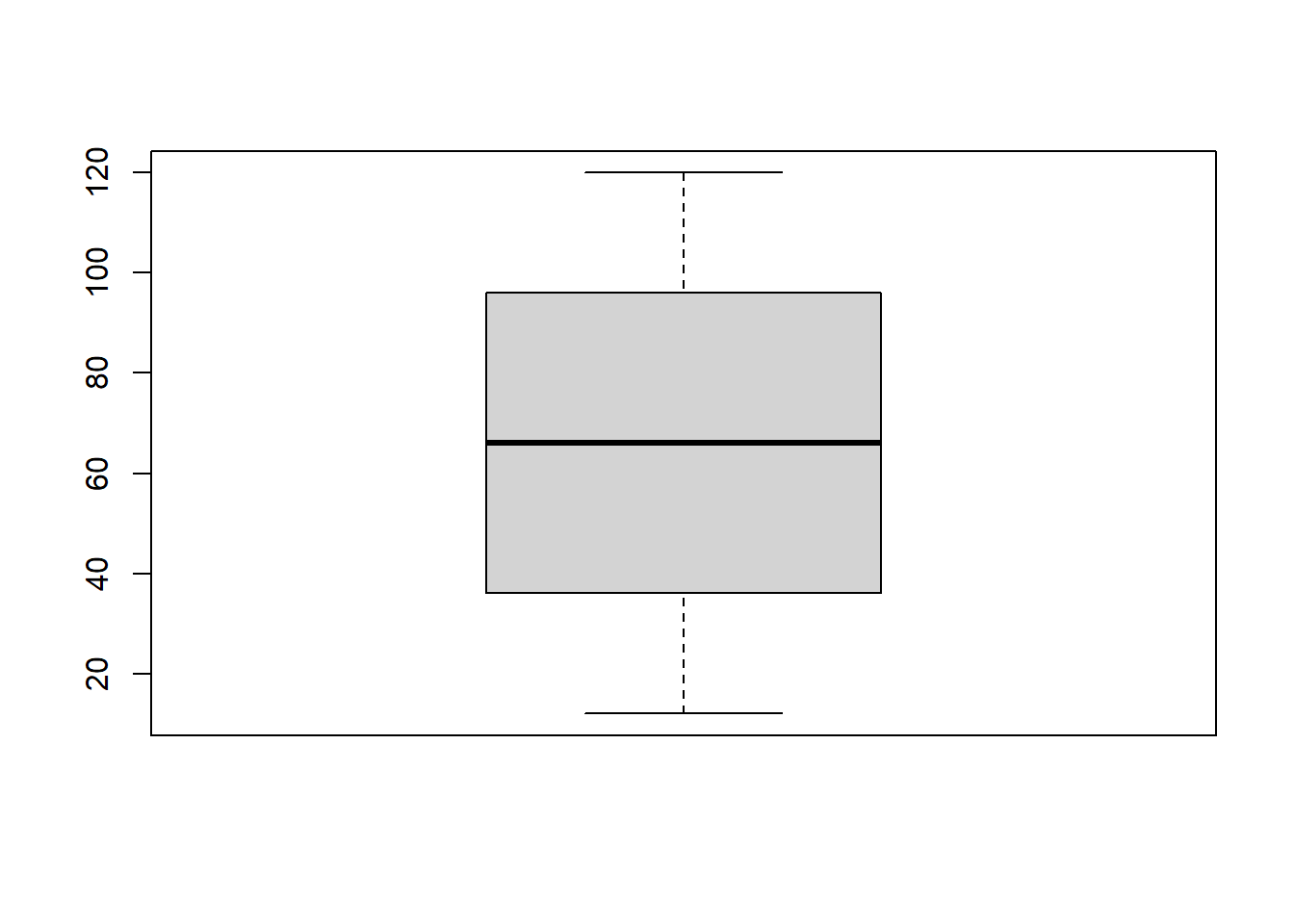# Unraveling Data Insights with R’s fivenum(): A Programmer’s Guide

rtip
Author

Steven P. Sanderson II, MPH

Published

July 25, 2023

# Introduction

As a programmer and data enthusiast, you know that summarizing data is essential to gain insights into its distribution and characteristics. R, being a powerful and versatile programming language for data analysis, offers various functions to aid in this process. One such function that stands out is `fivenum()`, a hidden gem that computes the five-number summary of a dataset. In this blog post, we will explore the `fivenum()` function and demonstrate how to leverage it for different scenarios, empowering you to unlock valuable insights from your datasets.

The five number summary is a concise way to summarize the distribution of a data set. It consists of the following five values:

• The minimum value
• The first quartile (Q1)
• The median
• The third quartile (Q3)
• The maximum value

The minimum value is the smallest value in the data set. The first quartile (Q1) is the value below which 25% of the data points lie. The median is the value below which 50% of the data points lie. The third quartile (Q3) is the value below which 75% of the data points lie. The maximum value is the largest value in the data set.

The five number summary can be used to get a quick overview of the distribution of a data set. It can tell us how spread out the data is, whether the data is skewed, and whether there are any outliers.

# How to use the fivenum() function in R

## Example 1. A Vector:

Let’s start with the basics. To compute the five-number summary for a vector in R, all you need is the `fivenum()` function and your data. For example:

``````# Sample vector
data_vector <- c(12, 24, 36, 48, 60, 72, 84, 96, 108, 120)

# Calculate the five-number summary
summary_vector <- fivenum(data_vector)

# Output the results
print(summary_vector)``````
``  12  36  66  96 120``

The `fivenum()` function will return the minimum, first quartile (Q1), median (Q2), third quartile (Q3), and maximum values of the vector. Armed with this information, you can easily visualize the dataset’s distribution using box plots, histograms, or other graphical representations.

## Example 2. With `boxplot()`:

Box plots, also known as box-and-whisker plots, are a fantastic visualization tool to display the distribution and identify outliers in your data. When combined with `fivenum()`, you can create insightful box plots with minimal effort. Consider this example:

``````# Sample vector
data_vector <- c(12, 24, 36, 48, 60, 72, 84, 96, 108, 120)

# Create a box plot
boxplot(data_vector)````````````# Calculate the five-number summary and print the results
summary_vector <- fivenum(data_vector)
print(summary_vector)``````
``  12  36  66  96 120``

By incorporating the `fivenum()` function, you can see the minimum, lower hinge (Q1), median (Q2), upper hinge (Q3), and maximum, represented in the box plot. This graphical representation helps in visualizing the spread of the data, presence of outliers, and skewness.

## Example 3. On a Column in a Data.frame:

Often, data is stored in data.frames, which are highly efficient for handling and analyzing datasets. To apply `fivenum()` on a specific column within a data.frame, use the `\$` operator to access the desired column. Consider the following example:

``````# Sample data.frame
data_df <- data.frame(ID = 1:5,
Age = c(25, 30, 22, 28, 35))

# Calculate the five-number summary for the "Age" column
summary_age <- fivenum(data_df\$Age)

# Output the results
print(summary_age)``````
`` 22 25 28 30 35``

By applying `fivenum()` on the “Age” column, you obtain the five-number summary, which reveals valuable information about the age distribution of the dataset.

## Example 4. Across Multiple Columns of a Data.frame Using `sapply()`:

To elevate your data analysis game, you’ll often need to summarize multiple columns simultaneously. In this case, `sapply()` comes in handy, allowing you to apply `fivenum()` across several columns at once. Let’s take a look at an example:

``````# Sample data.frame
data_df <- data.frame(ID = 1:5,
Age = c(25, 30, 22, 28, 35),
Salary = c(50000, 60000, 45000, 55000, 70000))

# Apply fivenum() on all numeric columns
summary_all_columns <- sapply(data_df[, 2:3], fivenum)

# Output the results
print(summary_all_columns)``````
``````     Age Salary
[1,]  22  45000
[2,]  25  50000
[3,]  28  55000
[4,]  30  60000
[5,]  35  70000``````

In this example, `sapply()` is used to calculate the five-number summary for the “Age” and “Salary” columns simultaneously. The output provides a comprehensive summary of these columns, enabling you to quickly assess the distribution of each.

# Conclusion

Congratulations! You’ve now unlocked the potential of R’s `fivenum()` function. By using it on vectors, data.frames, and even in conjunction with `boxplot()`, you can efficiently summarize data and gain deeper insights into its distribution and characteristics. Embrace the power of `fivenum()` in your data analysis endeavors and embark on a journey of discovery with your datasets. Don’t hesitate to explore further and adapt the function to your unique data analysis needs. Happy coding!